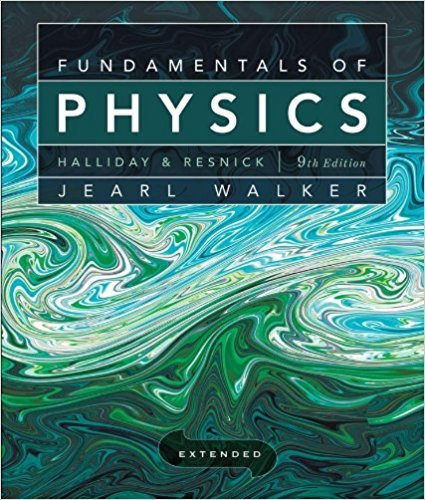×
×

# Solved: Figure 18-50 displays a closed cycle for p a gas.ISBN: 9780470469088 189

## Solution for problem 69 Chapter 18

Fundamentals of Physics Extended | 9th Edition

• Textbook Solutions
• 2901 Step-by-step solutions solved by professors and subject experts
• Get 24/7 help from StudySoup virtual teaching assistantsFundamentals of Physics Extended | 9th Edition

4 5 1 342 Reviews
22
1
Problem 69

Figure 18-50 displays a closed cycle for p a gas. The change in internal energy along path ea is -1601. The energy transferred to the gas as heat is 200 J along path ab, and 40 J along path be. How much work is done by the gas along (a) path abc and (b) path ab?

Step-by-Step Solution:
Step 1 of 3
Step 2 of 3

Step 3 of 3

##### ISBN: 9780470469088

Since the solution to 69 from 18 chapter was answered, more than 222 students have viewed the full step-by-step answer. The full step-by-step solution to problem: 69 from chapter: 18 was answered by , our top Physics solution expert on 12/27/17, 08:15PM. This textbook survival guide was created for the textbook: Fundamentals of Physics Extended, edition: 9. Fundamentals of Physics Extended was written by and is associated to the ISBN: 9780470469088. This full solution covers the following key subjects: . This expansive textbook survival guide covers 37 chapters, and 3413 solutions. The answer to “Figure 18-50 displays a closed cycle for p a gas. The change in internal energy along path ea is -1601. The energy transferred to the gas as heat is 200 J along path ab, and 40 J along path be. How much work is done by the gas along (a) path abc and (b) path ab?” is broken down into a number of easy to follow steps, and 56 words.

Unlock Textbook Solution